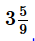Courses

# Test: Conversion Of Solids

## 20 Questions MCQ Test Mathematics (Maths) Class 10 | Test: Conversion Of Solids

Description
This mock test of Test: Conversion Of Solids for Class 10 helps you for every Class 10 entrance exam. This contains 20 Multiple Choice Questions for Class 10 Test: Conversion Of Solids (mcq) to study with solutions a complete question bank. The solved questions answers in this Test: Conversion Of Solids quiz give you a good mix of easy questions and tough questions. Class 10 students definitely take this Test: Conversion Of Solids exercise for a better result in the exam. You can find other Test: Conversion Of Solids extra questions, long questions & short questions for Class 10 on EduRev as well by searching above.
QUESTION: 1

### A solid iron rectangular block of dimensions 4.4 m, 2.6 m and 1 m is melted and cast into a hollow cylindrical pipe of internal radius 30 cm and thickness 5 cm. The length of the pipe will be​

Solution:

Given that:

Internal radius r=30 cm
Thickness w=5 cm
External radius R=30+5=35 cm

Let the length of pipe be h.

Here,

The volume of cuboid = Volume of a hollow cylinder

440×260×100=π(R2–r2)h

⇒440×260×100=π(352–302)h

⇒440×260×100=π(325)h

⇒h=11204

The length of the pipe h=11204 cm=112.04 m

QUESTION: 2

### A hemisphere of lead of radius 9 cm is cast into a right circular cone of height 72 cm. The radius of the base of cone is

Solution:

Make volume of cone = volume of hemisphere

QUESTION: 3

### An iron ball of radius 10.5 cm is melted and recast into small cones, each of radius 3.5 cm and height 3 cm. The number of cones is

Solution:
QUESTION: 4

The diameter of a sphere is 6 cm. It is melted and drawn into a wire of diameter 2 cm. The length of the wire is​

Solution:
QUESTION: 5

How many balls, each of radius 1 cm, can be made from a solid sphere of lead of radius 8 cm

Solution:
QUESTION: 6

How many coins 1.75 cm in diameter & 2 mm thick must be melted to form a cuboid 11 cm x 10 cm x 7 cm?​

Solution:
QUESTION: 7

The largest sphere is curved out of a cube of a side 7 cm. Find the volume of the sphere.​

Solution:
QUESTION: 8

How many coins 1.75 cm in diameter & 2 mm thick must be melted to form a cuboid 11 cm x 10 cm x 7 cm?

Solution:
QUESTION: 9

A hemispherical bowl of internal diameter 30 cm contains a liquid. The liquid is to be filled in cylindrical bottles of diameter 5 cm and height 6 cm. The number of bottles required to empty the bowl are​

Solution:
QUESTION: 10

A circular cylinder shaped container having diameter 24 cm and height 10 cm is full of ice-cream. The ice-cream is to be filled into cones of height 8 cm and diameter 4 cm, having a hemispherical shape on the top. The number of such cones which can be filled with ice-cream is​

Solution:
QUESTION: 11

A spherical shell of lead, whose external diameter is 18 cm, is melted and recast into a right circular cylinder, whose height is 8 cm and diameter 12 cm. The internal diameter of the shell is:​

Solution:
QUESTION: 12

The diameter of a copper sphere is 6 cm. The sphere is melted and recast into a wire. If the length of the wire is 36 cm, its radius is​

Solution:
QUESTION: 13

The number of lead balls each of radius1 cm, that can be formed from a sphere of radius 8 cm will be​

Solution:
QUESTION: 14

The largest possible sphere is carved out from a cube of 7 cm side. The volume of the sphere will be

Solution:
QUESTION: 15

The material of a cone is converted into the shape of a cylinder of equal radius. If the height of the cylinder is 5 cm, then the height of the cone is​

Solution:
QUESTION: 16

If a solid right circular cone of height 24 cm and base radius 6 cm is melted and recast in the shape of a sphere, then the radius of the sphere is​

Solution:
QUESTION: 17

How many balls, each of radius 1 cm, can be made from a solid sphere of lead of radius 8 cm.

Solution:
QUESTION: 18

A sphere of diameter 12 cm is dropped into a right circular cylindrical vessel partly filled with water. If the sphere is completely submerged in water, the water level in the cylindrical vessel rises bycm, the radius of the cylindrical vessel will be​

Solution:
QUESTION: 19

A solid consists of a circular cylinder with an exact fitting circular cone placed at the top. The height of the cone is h. If the total volume of the solid is 3 times the volume of the cone, then the height of the circular cylinder is

Solution:
QUESTION: 20

2 cubes of volume 64 cm3 are joined end to end. Find the surface area of resulting cuboid

Solution:

Let ‘a’ be the length of a side of a cube. Then

Volume of one cube = 64 cm3

⇒   n3 = 65 cm3

⇒   n = 4 cm

On joining the cubes, a cuboid is formed.

Then The length of the resulting cuboid (l) = 2

The breadth of the resulting cuboid (b) = a cm

The thickness of the resulting cuboid (h) = a cm

Now,

Surface area of the resulting cuboid

= 2(lb + bh + hl)

= 2 (2a. a + a. a + a. 2a)

= 2 (2a2 + a2 + 2a2)

= 2 (5a2) = 10a2 = 10 (4)2

= 160 cm.